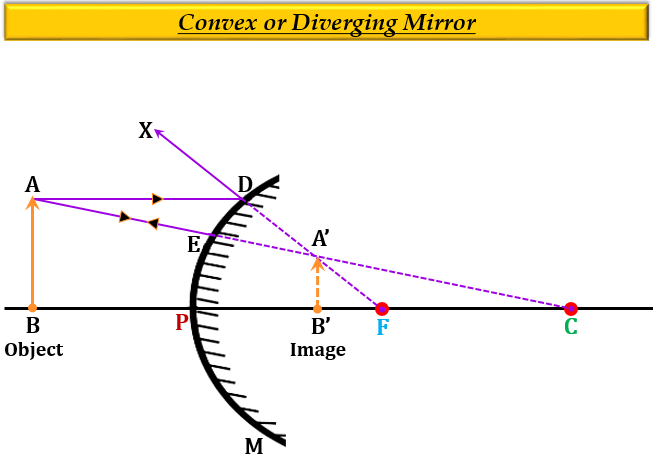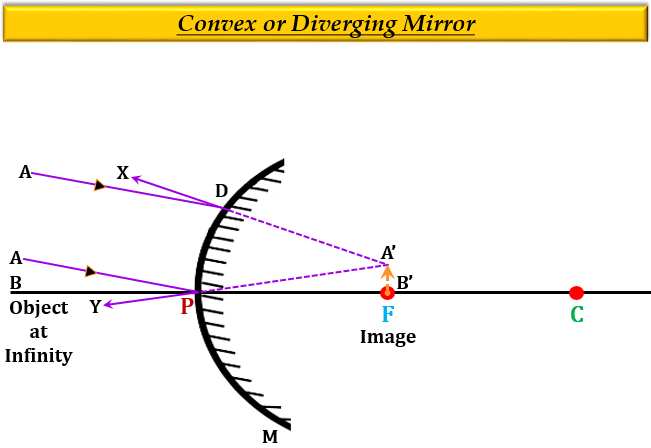# (a) Draw a labelled ray diagram to show the formation of image of an object by a convex mirror. Mark clearly the pole, focus and centre of curvature on the diagram.(b) What happens to the image when the object is moved away from the mirror gradually?(c) State three characteristics of the image formed by a convex mirror.

(a) Formation of the image by a convex mirror when the object is placed anywhere between the pole and infinity.Formation of the image by a convex mirror when the object is at infinity (large distance).(b) When the object is moved away from the mirror gradually, the size of the image decreases.

(c) Three characteristics of the image formed by a convex mirror are:

1. virtual (behind the mirror).

2. erect (upright).

3. diminished (smaller than the object).

Updated on: 10-Oct-2022

180 Views